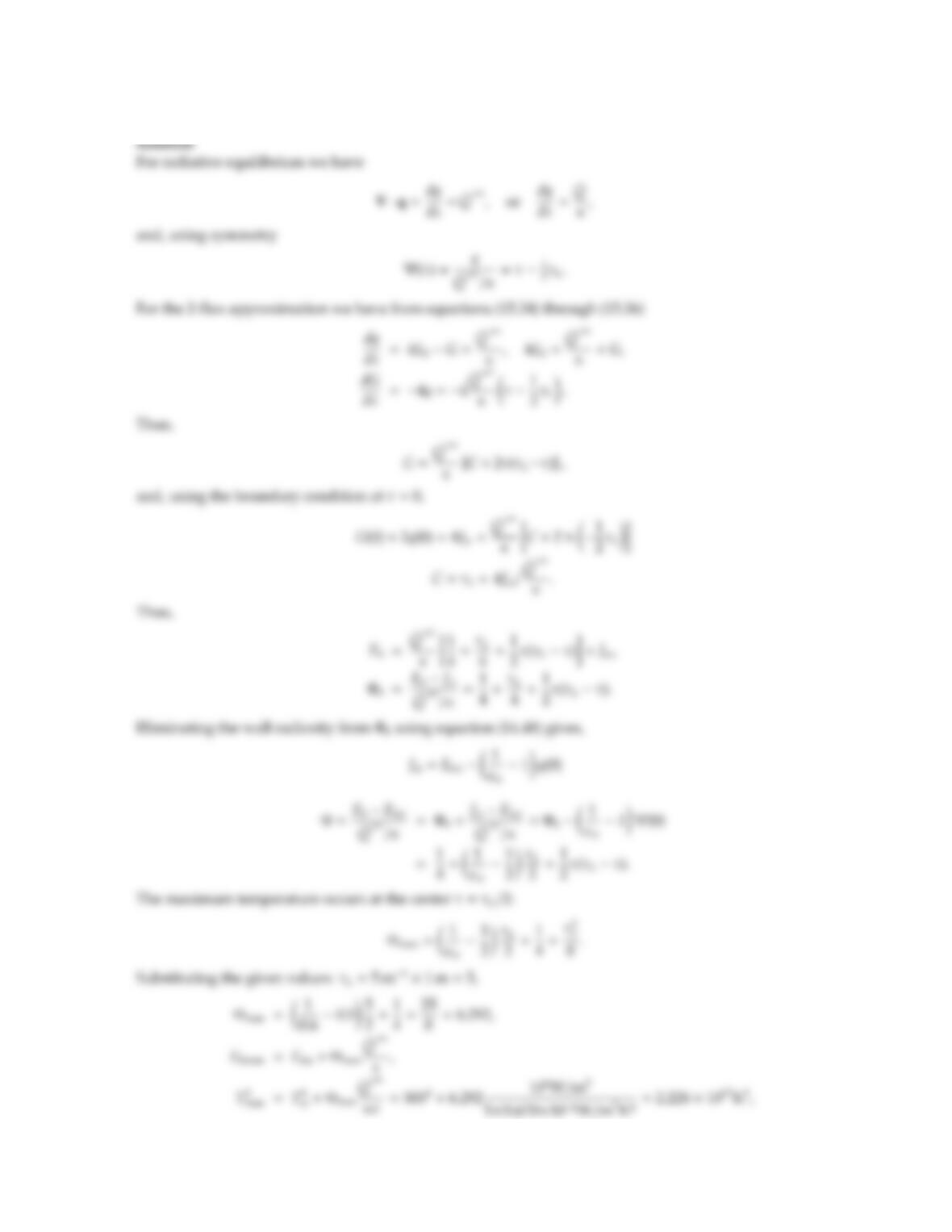# 978-0123869449 Chapter 15 Part 1

Document Type
Homework Help
Book Title
Authors
Michael F. Modest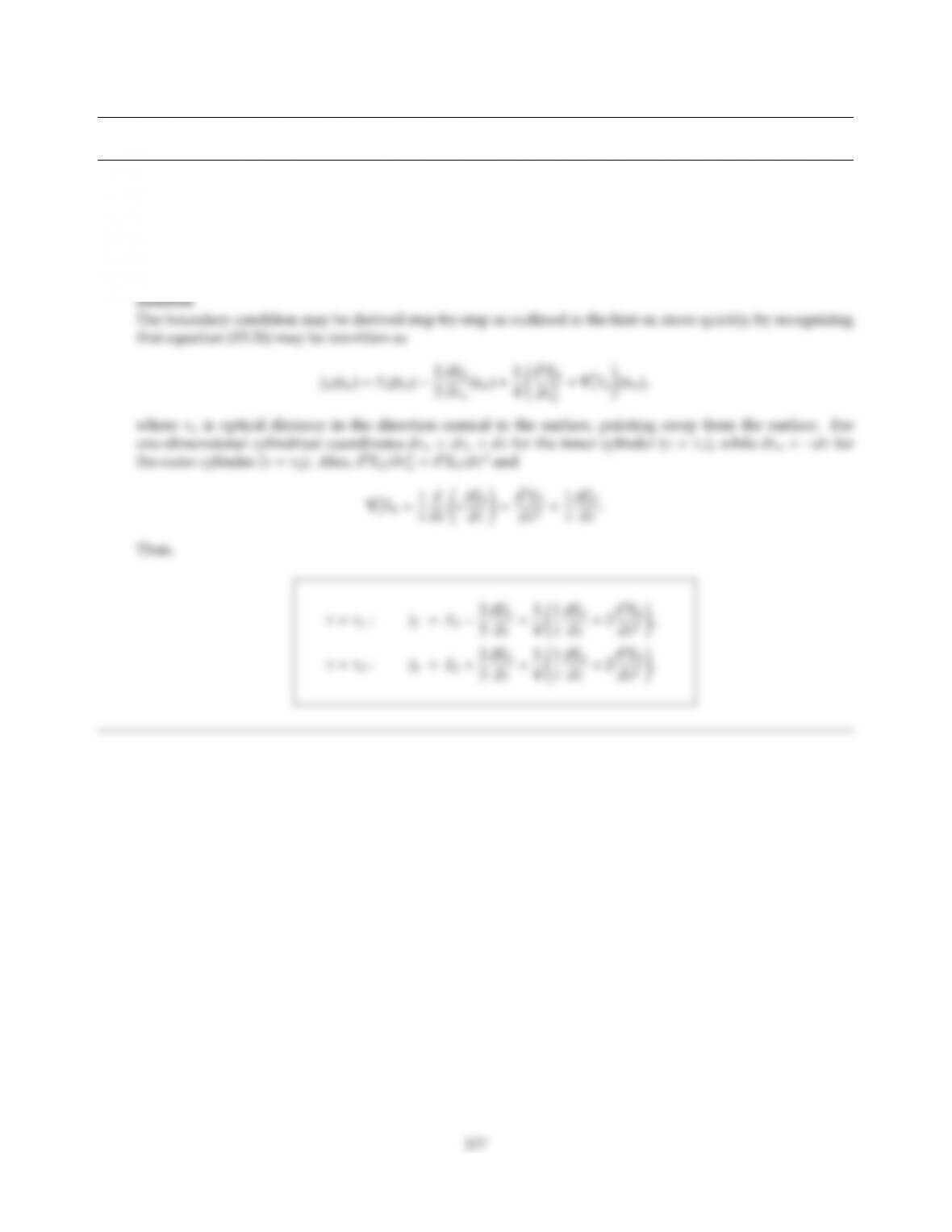CHAPTER 15
15.1 Derive the jump boundary condition for the diusion approximation, equation (15.26), for the case of concen-
tric cylinders. Assume the heat transfer to be one-dimensional (only radial, no azimuthal or axial dependence).
Hint: Introduce a local Cartesian coordinate system at a point at the boundary, express any other r-location
within the medium in terms of x,y,z, and transform the derivatives in equation (15.26) to r-derivatives; finally
let x,y,zgo to zero (since the derivatives at the boundary are needed).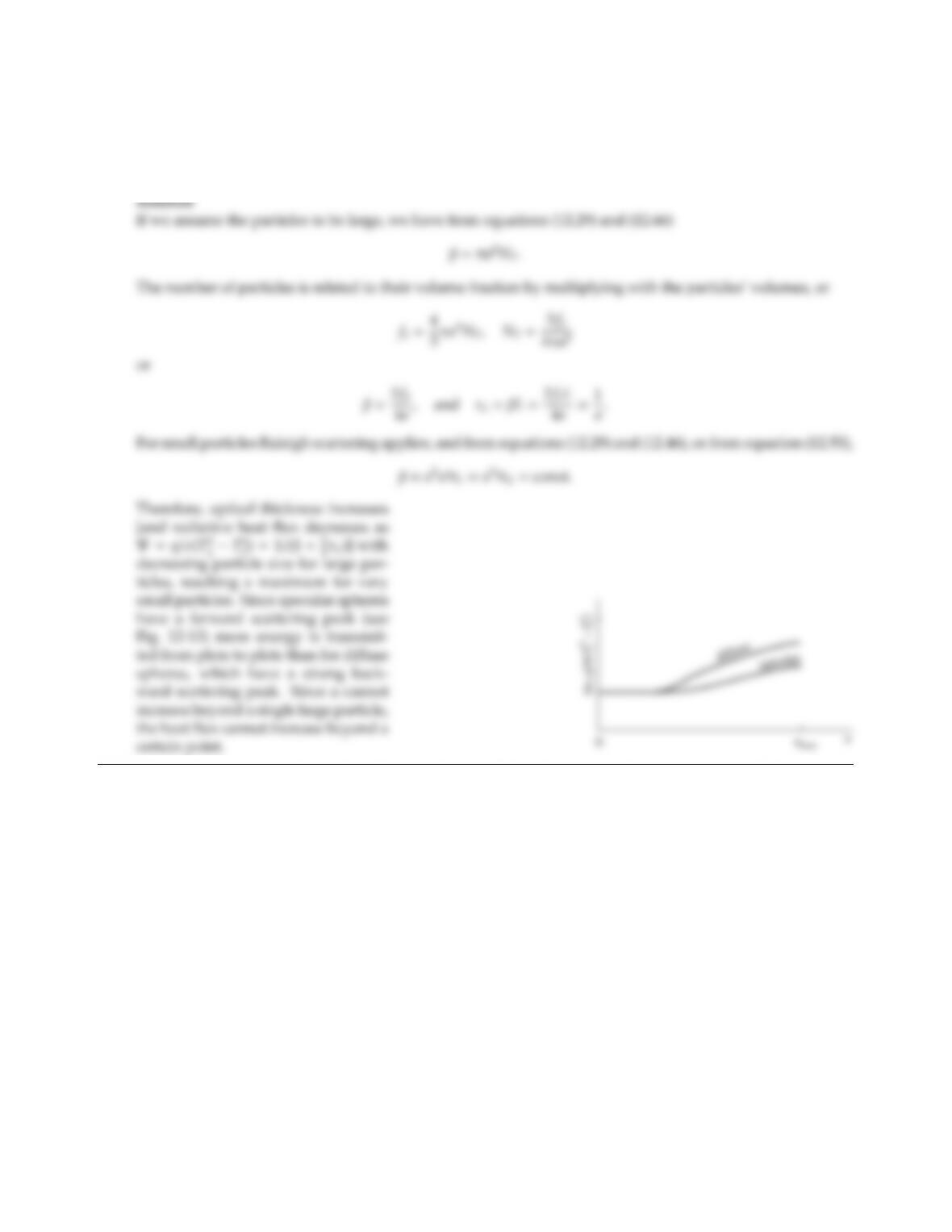15.2 The gap between two parallel black plates at T1and T2, respectively, is filled with a particle-laden gas.
from two dierent materials (one a specular reﬂector, the other a diuse reﬂector, both having the same ǫ).
Sketch nondimensional heat ﬂux Ψ = q(T4
1T4
2) vs. particle size (but keeping volume-fraction constant).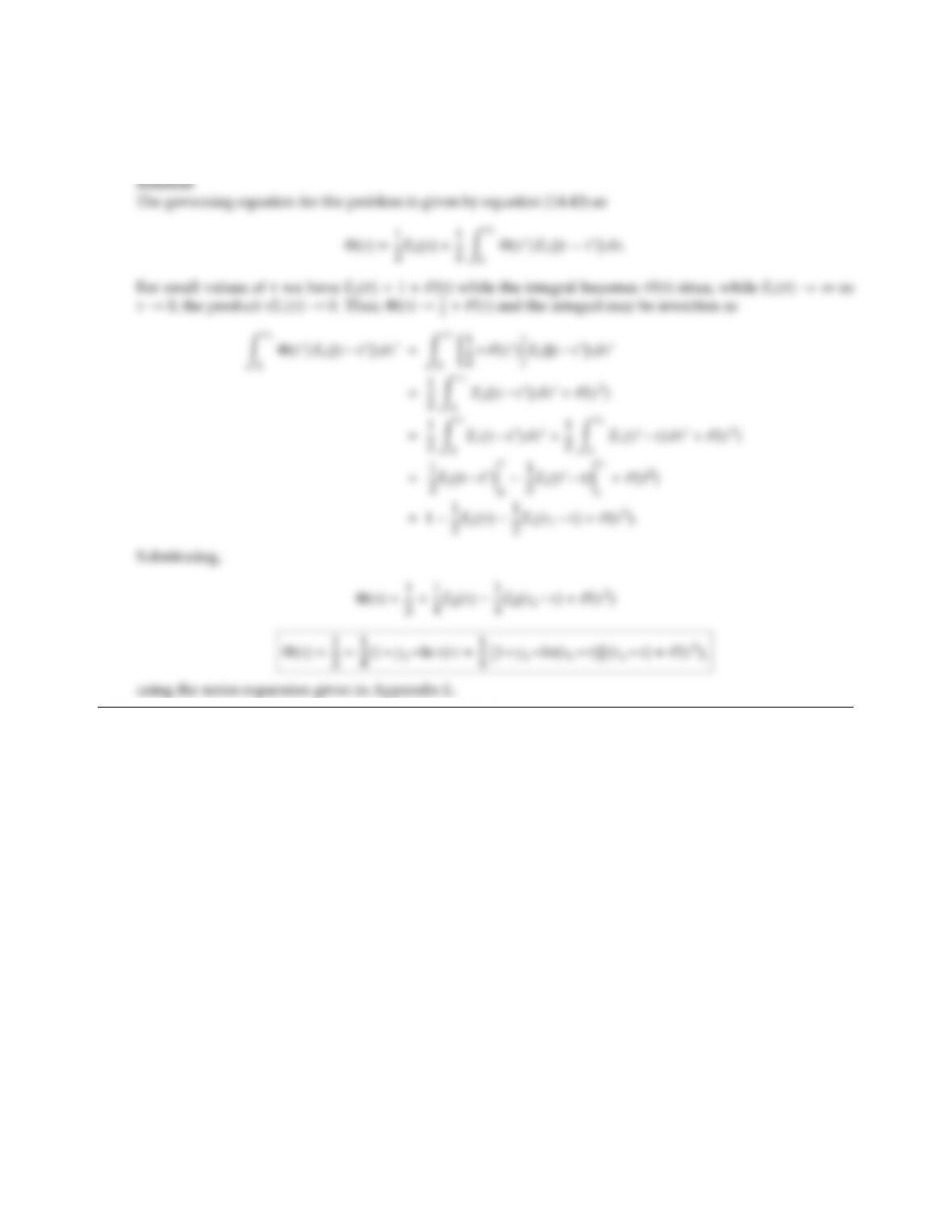CHAPTER 15 379
15.3 Consider radiative equilibrium of a gray, absorbing, emitting, and isotropically scattering medium contained
between two isothermal, gray-diuse, parallel plates spaced a distance Lapart. Determine the nondimen-
sional temperature variation within the medium, Φ = (σT4J2)/(J1J2) for the optically thin case (τL1).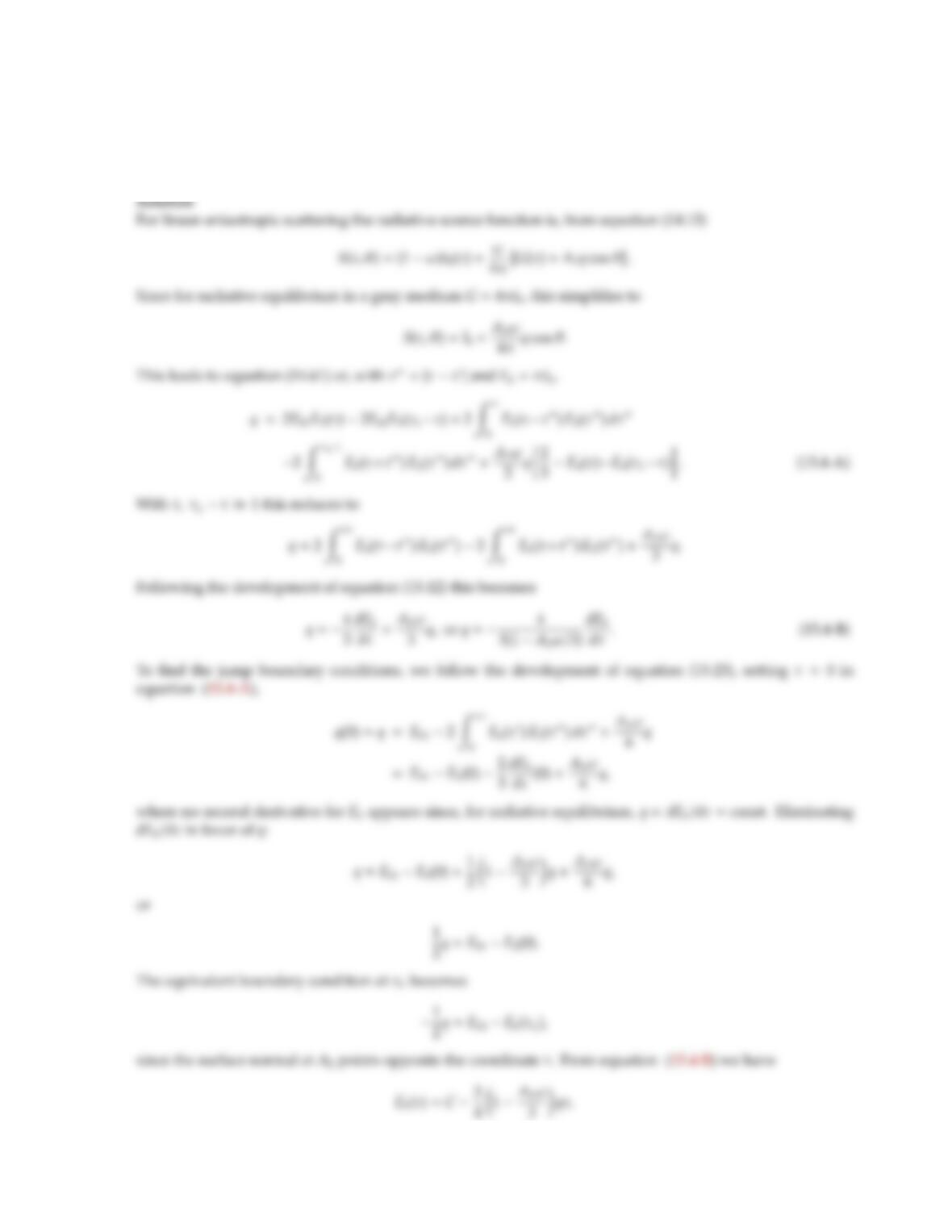15.4 Consider a gray, absorbing-emitting, linear-anisotropically scattering medium at radiative equilibrium. The
medium is confined between two parallel, isothermal, black plates (at temperatures T1and T2). Determine
an expression for the radiative heat ﬂux between the two plates using the diusion approximation with jump
boundary conditions.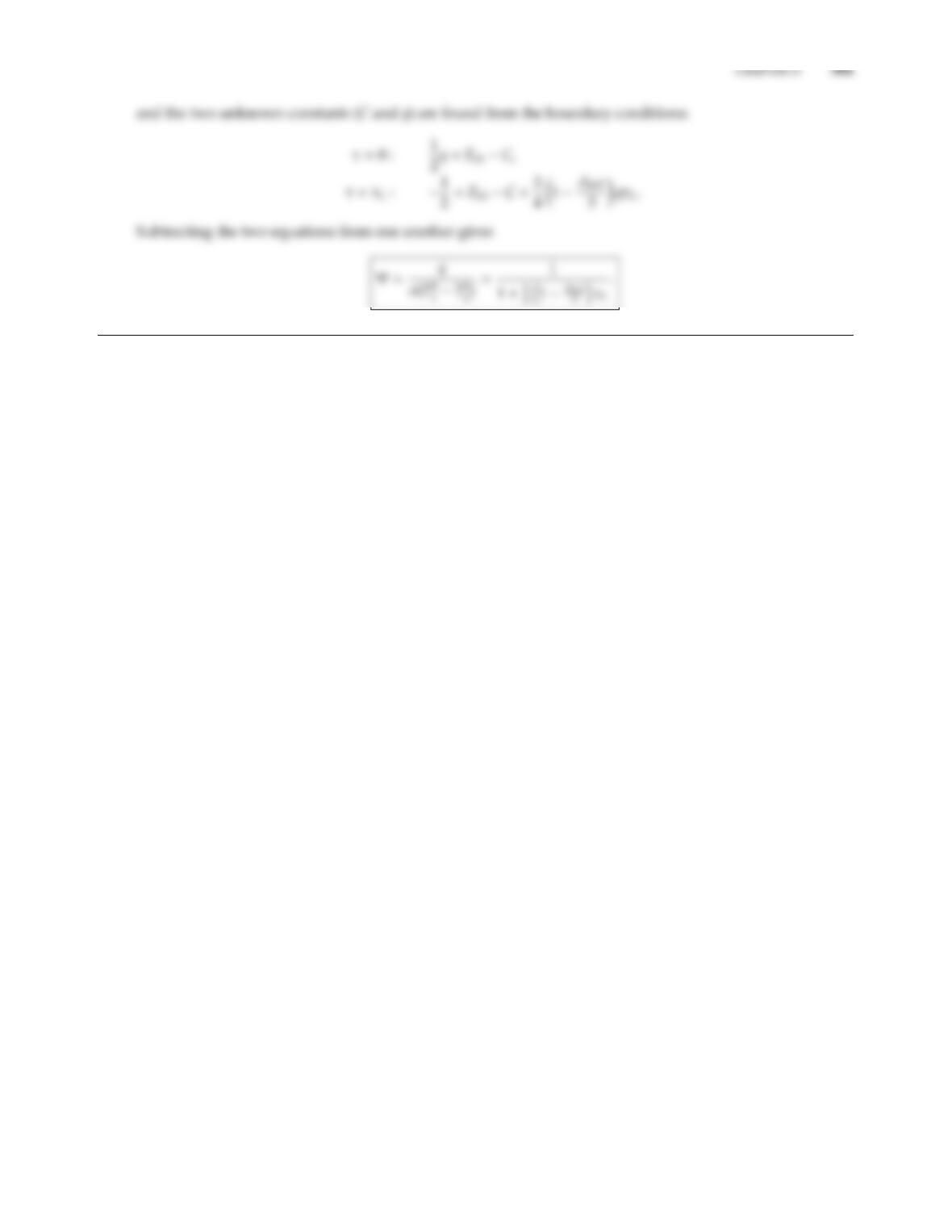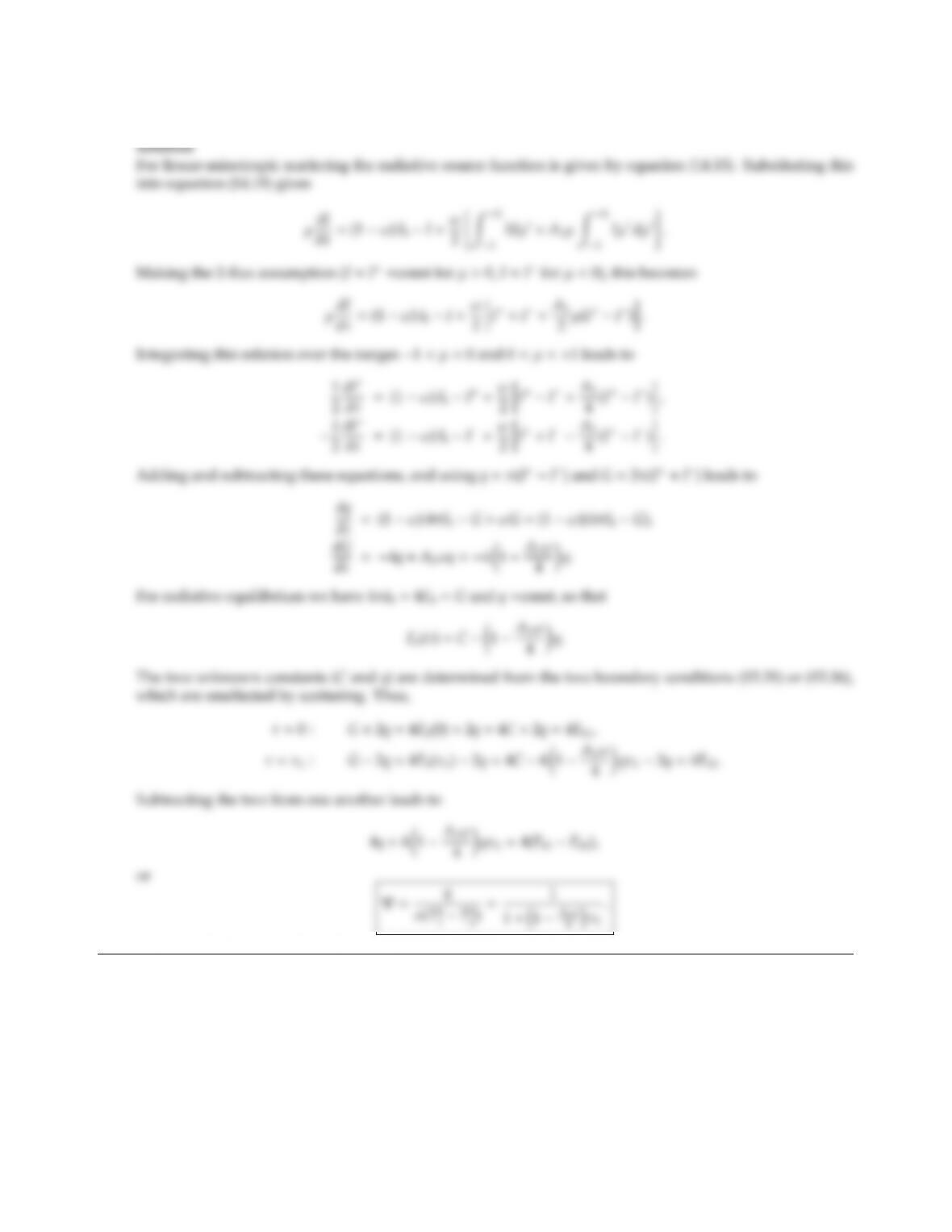15.5 Do Problem 15.4 using the Schuster-Schwarzschild (2-ﬂux) approximation.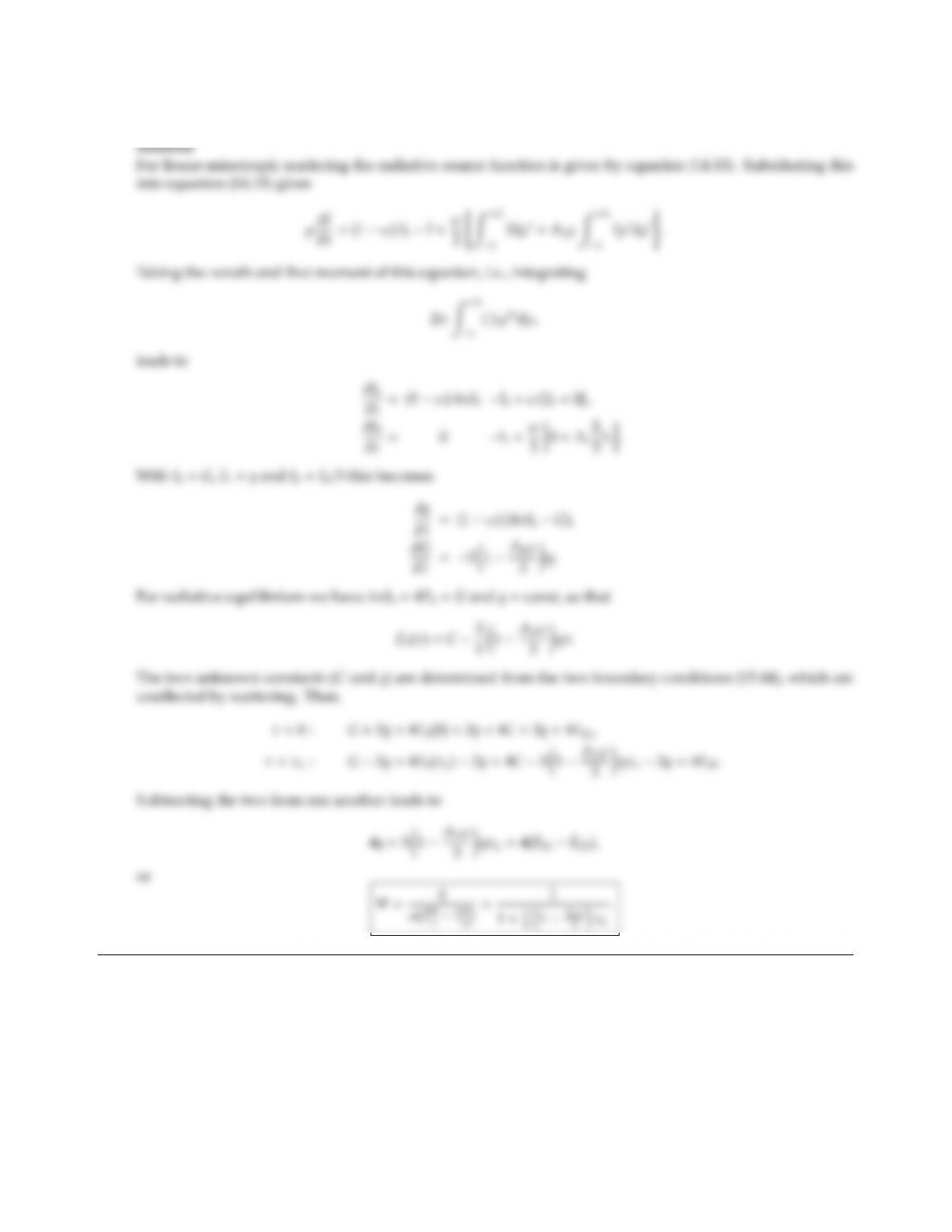CHAPTER 15 383
15.6 Do Problem 15.4 using the Milne-Eddington (dierential) approximation.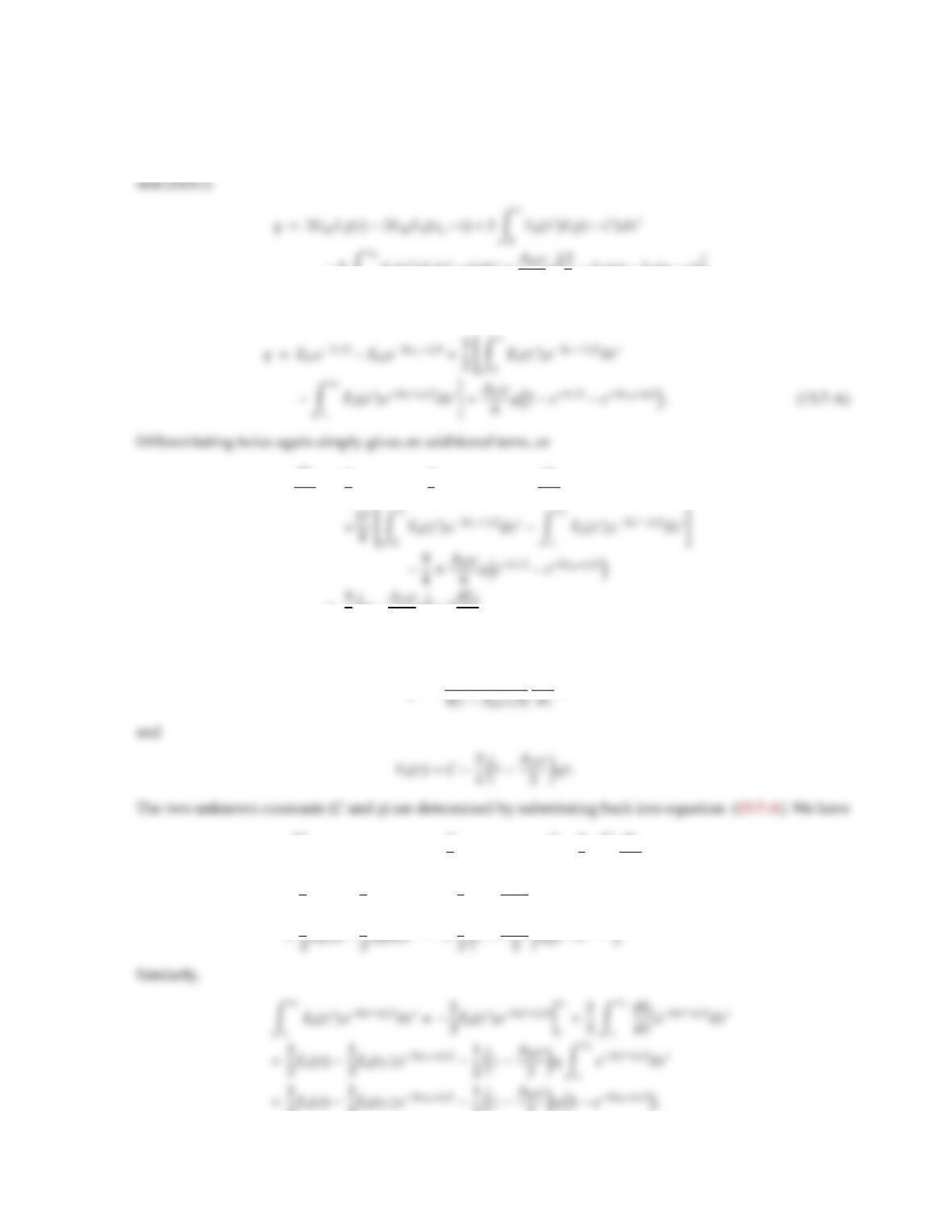15.7 Do Problem 15.4 using the exponential kernel approximation method.
Solution
2ZτL
τ
Eb(τ)E2(ττ)dτ+A1ω
2q2
3E4(τ)E4(τLτ).
Using the kernel approximation this leads to equation (15.48) with an additional term,
d2q
dτ2=9
4Eb1e3τ/29
4Eb2e3(τL
τ)/2+3dEb
dτ
=9
4qA1ω
3q+3dEb
dτ.
Since, for radiative equilibrium, q=const and d2q/dτ2=0 this implies
q=4
dEb
0
Eb(τ)e3(ττ)/2dτ=2
3Eb(τ)e3(ττ)/2
τ
02
3Zτ
0
dEb
dτe3(ττ)/2dτ
=2
3Eb(τ)2
3Eb(0) e3τ/2+1
21A1ω
3qZτ
0
e3(ττ)/2dτ
3Eb(τ)2
3Eb(τL)e3(τL
31A1ω
3q1e3(τL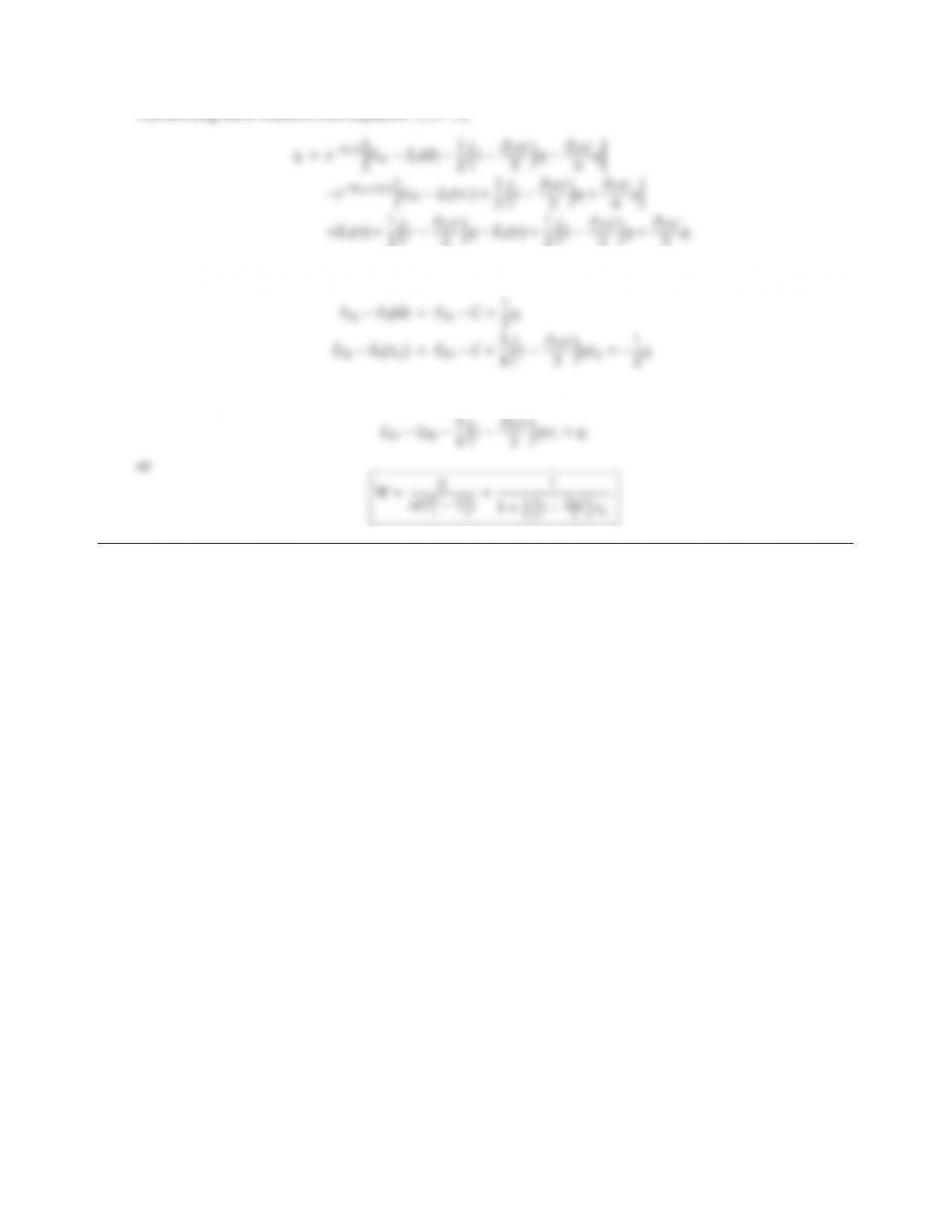CHAPTER 15 385
Substituting these relations into equation (15.7-A)
Note that the terms without exponentials cancel each other; similarly, the terms in brackets must be zero, i.e.,
Subtracting the two from one another leads to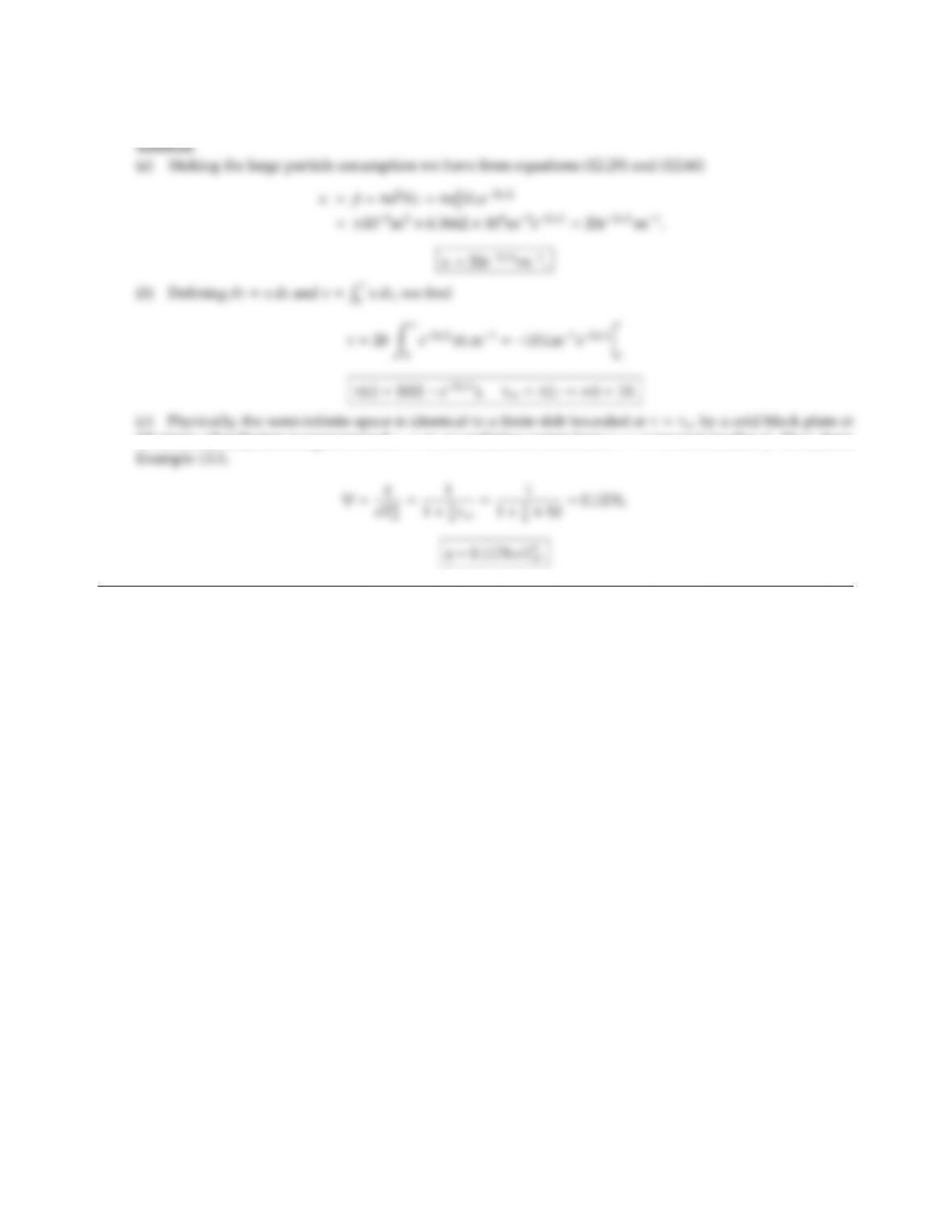15.8 Do Problem 14.7 using the Milne-Eddington (dierential) approximation.
0 K (since all radiation escapes toward z→ ∞, no radiation enters from z toward smaller z). Thus, from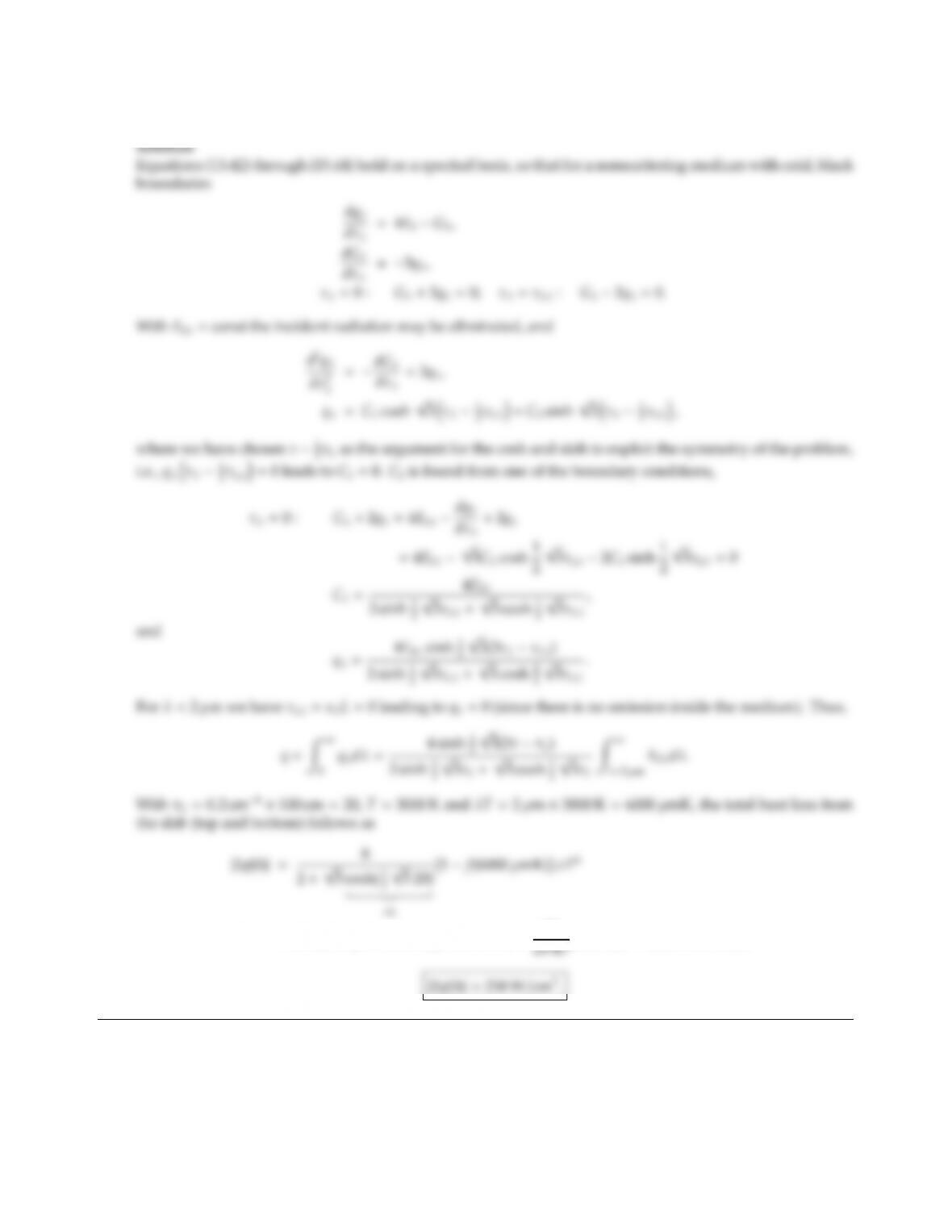CHAPTER 15 387
15.9 Do Problem 14.11 using the Milne-Eddington (dierential) approximation.
=2.1436 ×[1 0.73778] ×5.670 ×108W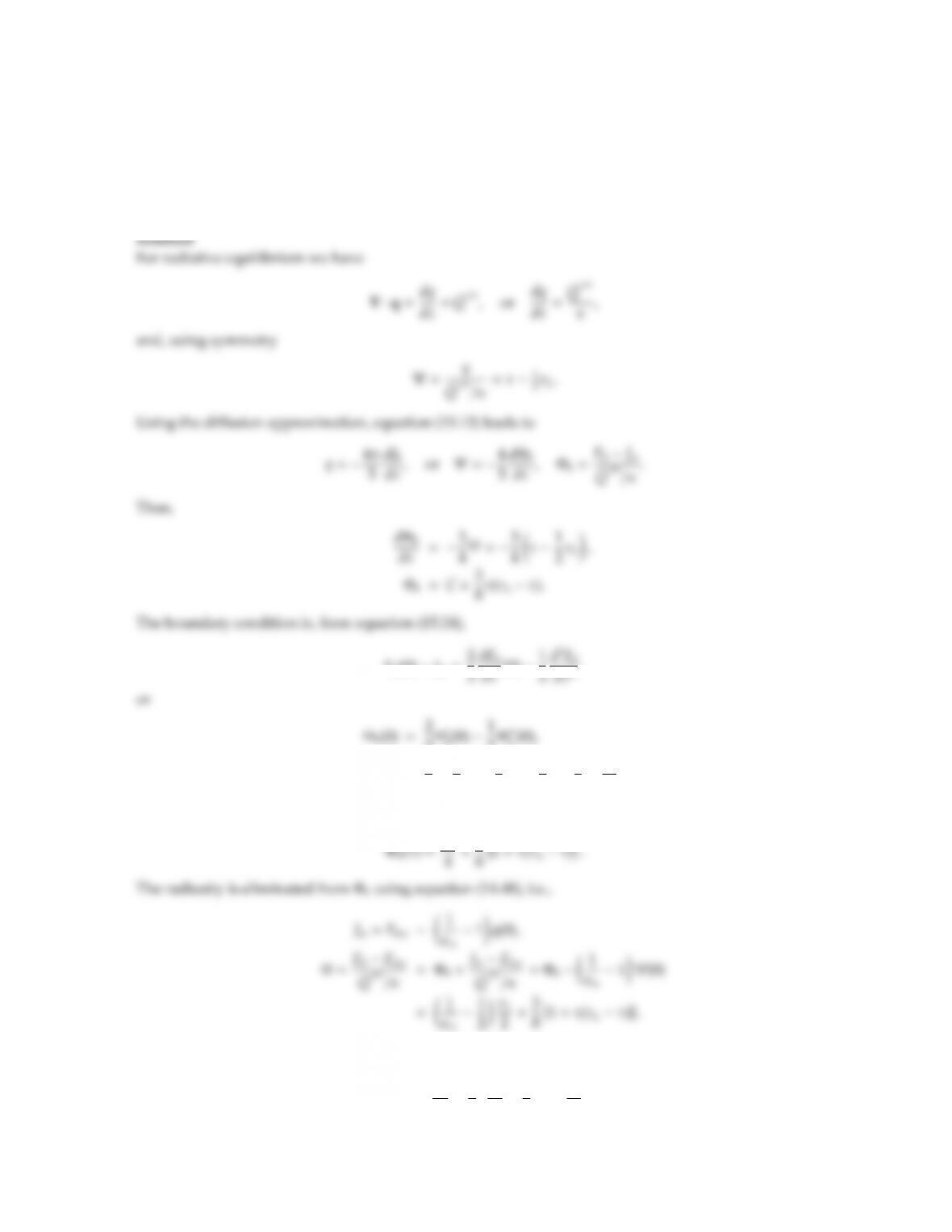15.10 Consider a space enclosed by infinite, diuse-gray, parallel plates 1 m apart filled with a gray, nonscattering
medium (κ=5 m1). The surfaces are isothermal (both at Tw=500 K with emittance ǫw=0.6), and there
is uniform and constant heat generation within the medium per unit volume, ˙
Q′′′ =106W/m3. Conduction
and convection are negligible such that ·q=˙
Q′′′. Determine the radiative heat ﬂux to the walls as well
as the maximum temperature within the medium, using the diusion approximation with jump boundary
conditions.
Eb(0) Jw=2
3Φ
b(0) 1
2Φ′′
b(0),
C=2
3×3
8τL1
2×3
4=3
8+τL
4.
Thus,
The maximum temperature occurs at the center, τ=τL/2:
Φmax =1
ǫw1
2τL
2+3
8 1+τ2
L
4!.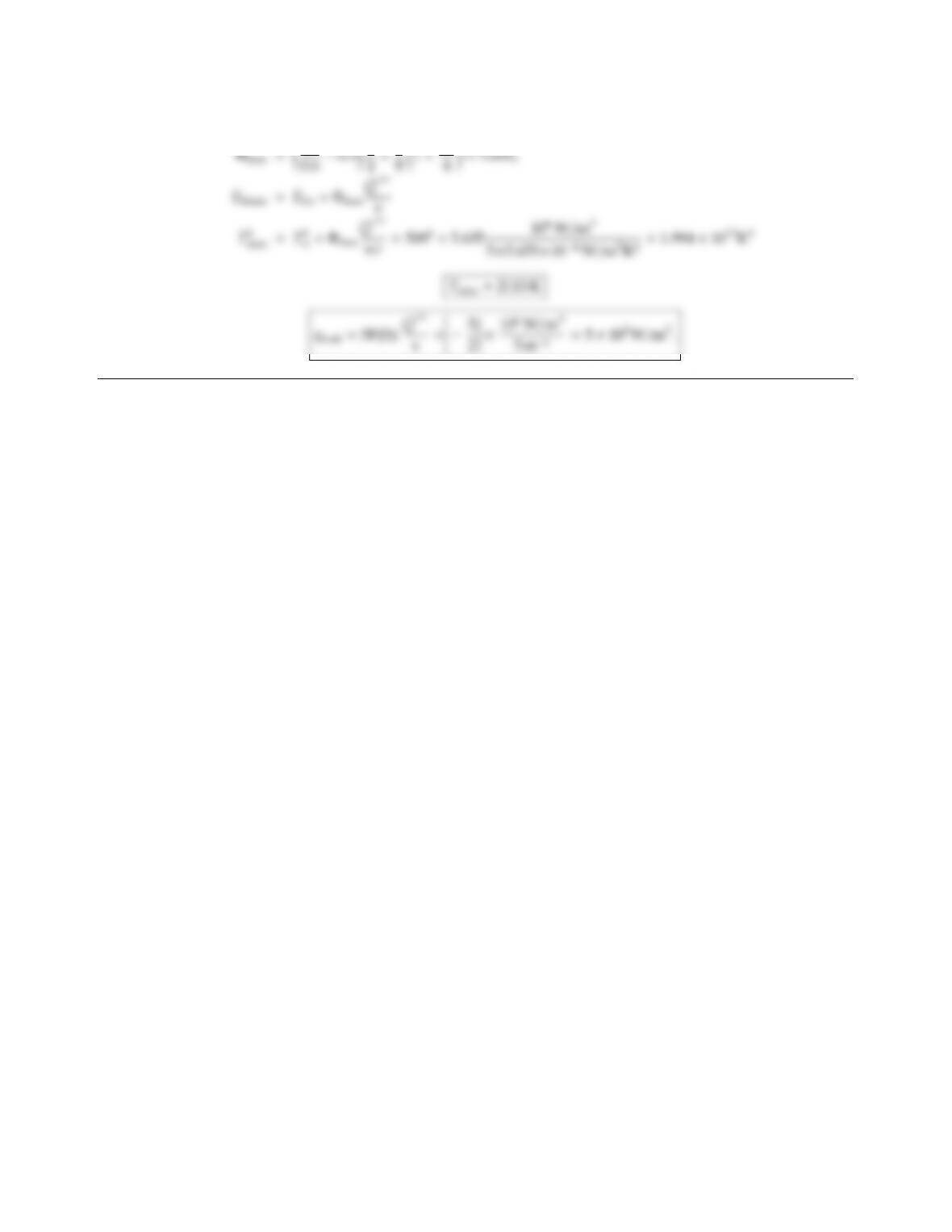CHAPTER 15 389
Substituting the given values: τL=5 m1×1 m =5,